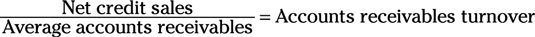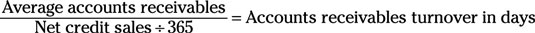##### Corporate Finance For Dummies, 2nd EditionThe accounts receivables turnover metrics are used by companies to determine whether they’re collecting the money that customers owe them. To find out exactly how well a company is at making sales that will be collected in the future and how well the company is at collecting the money that people owe it, the company can use the accounts receivables turnover metric, which it calculates like this:To put this equation to use, follow these steps:

1. Find net credit sales on the income statement.

2. Use the balance sheets of the current year and the previous year to calculate the average gross receivables: Add the accounts receivables from both years and divide that number by 2.

3. Divide net sales by the answer from Step 2 to get the accounts receivables turnover.

This metric is called a turnover because it measures the number of times the average value of a company’s accounts receivables turns over — in other words, the number of times the accounts receivables have been collected as sales and started fresh with brand new receivables.

Of course, the company is still acquiring receivables, so the receivables account is never depleted; however, the average value can still be collected as sales. If the number is very low, then the company may be having a difficult time collecting the money it’s owed.

Companies can use the accounts receivables turnover in days liquidity metric to calculate the number of days it takes them to collect an amount equivalent to the average total amount they're owed. As a company collects the money it’s owed, the total amount the company is owed on average goes up or down depending on how quickly it collects its money.

The accounts receivables turnover in days metric looks like this:Follow these steps to use this equation:

1. Use the balance sheets of the current year and the previous year to calculate the average gross receivables: Add the accounts receivables from both years and divide that number by 2.

2. Find net sales near the bottom of the income statement for the current year.

3. Divide net sales by 365.

The answer you get is the average number of sales the company is making per day in the current year.

4. Divide the answer from Step 1 by the answer from Step 3 to get the number of days it takes to collect as sales a total value that’s equivalent to the average amount of money the company is owed as receivables.